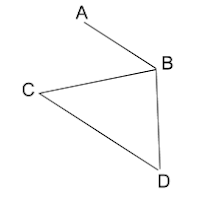### Day 32: Types of Graphs

Hello Dear Students,
Hope you all are doing good,

Aaj hum graph types ke baare mein study karenge.

So, let's get started...

Graph is a diagram or drawing which is collection of vertices(nodes) and edges. The various types of graphs are as follows-

• DIRECTED GRAPH - Directed graph means jis graph mein edges ki direction ho, means how edges moves from one vertex to another and what is the direction. For example,
In above graph, it is a directed graph because each edge shows a direction. e1 edge B to A ki direction mein point kar raha hai, e2 from B to C, and e3 from C to D. Edges are also known as Arcs. B to C move kar raha hai e2, then B is tail and C is head. e3 is from C to D, then C is tail and D is head.

• UNDIRECTED GRAPH - Undirected graph means graph jisme directions na ho and not a directed graph. For example, the below graph is undirected graph jisme edges ki directions nahi hai.• NULL GRAPH - Null graph is the graph jisme vertices hoti and but vertices ko connect karne ke liye edges nahi hote. In simple words, null graph is tha graph in which all the vertices are isolated.
• MULTIGRAPHS - Multigraph wo graph hota hai jisme parallel edges and loops hoti hain and mainly, multigraph is graph in which sum of degree of vertices is twice the number of edges.For example,

In above multigraph, sum of degree of vertices = 2 * number of edges.
Number of edges = 6
Degree of vertices,
d(A)=3
d(B)= 3
d(C)= 2
d(D)= 4
So, total sum of degree of vertices = 3+3+2+4 = 12
Number of edges = 6,
So, 12=2*6, 12=12, which is true.

• FINITE GRAPH - Finite graph means jisme finite number of vertices and edges hon. Finite number of vertices agar hongi then automatically finite number of edges honge. Finite number means which is countable and not infinity.
• TRIVIAL GRAPH - Trivial graph means wo graph jisme only vertex ho and no edges. It is also called single point graph because ek hi vertex hoti hai and koi bhi edges nahi hote.
• DISCRETE GRAPH - Discrete graph wo graph hota hai jisme n number of vertices hote hain but koi bhi edges nahi hote. Isme multiple vertices hote hain but without any edges.
The above are 2 complete graphs where each node connects to each other node.

Above are some regular graphs jisme every vertex have same degree, 1st have degree 1, all others have degree 2 of vertices.

• PLANAR GRAPH - Planar graph is graph in which no 2 edges intersects with each other. Graph jisme ek edge doosri edge ko intersect na kare wo graph planar graph hota hai. Above all are planar graphs.
• BIPARTITE GRAPH - Bipartite graph wo graph hota hai jisme total vertices ke 2 partitioning vertices honge such that edge ka ek point ek partition mein hoga and doosra point doosre partition mein hoga. For example,

Above graph is bipartite graph, agar hum A,C, and E ko ek partition assume karte hain(P1) and B,D, and F ko doosra partition assume karte hain(P2), then e1 ka ek point P1 mein hai and doosra point P2 mein, and aise hi A to D means e2 ka ek point A P1 mein hai and doosra point D P2 mein hai. So, it is a bipartite graph. But below is non bipartite graph.
The above graph is non bipartite graph because agar hum ise 2 partitions mein divide karenge, A and C as P1 and B and D as P2, then edges ke end P1 mein bhi hai and P2 mein bhi, but in bipartite graph only 1 edge point should be in P1 and other should be in P2. Hope you understand this because it is an important topic.

• EULER GRAPH - Euler graph is the graph which contains either euler path or euler circuit. Now,
Euler path is the path which visits every edge of graph exactly once. Means ek aisa path jo ki har ek edge ko visit kare sirf ek baar.
Euler Circuit is euler path whose start and end vertices are same.
The above is Euler path as 5,3,2,1,4. In this each edge is visited exactly once.
And this is Euler circuit in which Euler path start and end vertex is same. If we visit Euler path means every edge in once. 5,3,2,1,4,6,5. Here, every edge is visited exactly once and the start and end vertex is same means 5 to 5.

• HAMILTONIAN GRAPH - Hamiltonian graph is the graph which contains either Hamiltonian path or Hamiltonian circuit. Now,
Hamiltonian path is the path that visits every vertex of the graph exactly once. Means graph jisme har ek vertex ko only one time visit kiya jaye.
Hamiltonian circuit is the circuit in which starting and ending vertex is same and forms Hamiltonian path.

Above is hamiltonian circuit which have path 1,2,3,4,5,1. In this, each vertex is visited and starting and ending vertex is same.

Best of Luck Students,
Do visit our website regularly for more content and for daily tests.

Regards,
UGC NET EXPERTS